# Kerala Syllabus 7th Standard Maths Solutions Chapter 13 New Numbers

You can Download New Numbers Questions and Answers, Activity, Notes, Kerala Syllabus 7th Standard Maths Solutions Chapter 13 help you to revise complete Syllabus and score more marks in your examinations.

## Kerala State Syllabus 7th Standard Maths Solutions Chapter 13 New Numbers

### New Numbers Text Book Questions and Answers

Negative temperature Textbook Page No. 178

Haven’t you seen the newspapers and TV giving daily temperatures at various places in the country? In many parts of northern India, the temperature is below zero during winter.
The temperature at which water freezes to ice is taken as zero degree Celsius (0°C). Temperatures below this are taken as -1°C, -2°C and so on.A column of mercury within a glass tube expands and rises when the temperature is increased, it contracts and falls when the temperature is increased, it contracts and falls when the temperature is low. Temperature is measured using such a device. In such thermometers used in cold countries, numbers below zero are also marked.
The thermometer in the picture shows a temperature between -20° C and -15° C.Colder and Colder

The coldest place in india is Dras, a town in the Kargil district of Kashmir. Temperatures as low as -60 °C has been recorded there.The coldest place in the world is the continent of Antartica.The lowest temperature in the world was recorded here : -89 °C

Limit of coldness Textbook Page No. 180

Considering the whole universe we know of, the lowest temperature is found in the star cluster named Boomerang Nebula, about fifty quadrillion (5 × 1016) kilometres from the earth. It is -272.15°C.This is the lowest temperature recorded in nature. Still lower temperatures are produced in labs.

According to the laws of physics, temperature of -273.15°C or below is impossible, either in nature or in labs.Negative Money

Even as early as the seventh century, negative numbers were used in India to denote debts in financial transactions. This practice continues to the day.

For example, many people use a prepaid scheme in their mobile phone connections, in which a certain amount of money is paid in advance. This gets reduced on usage. The subscriber can see at any time how much is left in the account. Even after the advance is exhausted, he can use the phone for some more time. The amount left will be shown as – 2 rupees or – 3 rupees then.
It means this amount will be deducted when the next advance is paid.

Negative floors Textbook Page No. 182

To go from one floor to another in tall buildings, a lift is used.
It has a panel of numbered buttons and we can get to a floor by pressing its number. The picture shows such a panel:Why are there negative numbers like -1 and -2 in it?
It means that the building has some floor below the ground level with the one just below the ground level named -1, the one below that -2 and so on.Changing direction

In discussing motion along a straight line, distances in one direction from a fixed point are often denoted by positive numbers and distances in the opposite direction by negative numbers.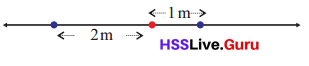In the picture above, distances to the right of the red dot are taken positive and to the left are taken negative.

If from this point, 3 metres to the right and then 5 metres to the left is travelled, where would be the final point reached? To the left or right of the point? How far away from it? This we can write as,
3 – 5 = – 2
What if 5 metres to the left is travelled first and then 3 metres to the right?
– 5 + 3 = – 2
5 metres to the left and then again 3 metres to the left?

Distance travelled = – 8 meters.

Explanation:
Distance to the left travelled first = 5 m.
Distance to the left travelled later = 3 m.
Distance travelled = Distance to the left travelled first + Distance to the left travelled later
= – 5 – 3
= -8 meters.

Speed Math Textbook Page No. 184

When an object is thrown up from the earth, it goes higher and higher with its speed decreasing every instant. When the speed becomes zero, it starts falling down. In the return journey, the speed increases, till it hits the ground.

If it is thrown directly upwards, the speed decreases by 9.8 m/s every second.
For example, if an object is thrown directly up with a speed of 49 m/s, then after 1 second, the speed becomes 49 – 9.8 = 39.2 m/s;
After 2 seconds, 49 – (2 × 9.8) = 29.4 m/s.
After 5 seconds, the speed becomes.
49 – (5 × 9.8) = 0
Thereafter, it falls down with speed increasing by 9.8 m/s every second.
What is the speed 7 seconds after throwing? Then, it has been falling for 7 – 5 = 2 seconds.
So the speed has increased by 2 × 9.8 m/s from 0; that is 19.6 m/s.

We can write these in shorter form using algebra. What is the speed t seconds after throwing?
If t <5, then the speed is 49 – 9.8 tm/s. And for t > 5? It has been falling for t – 5 seconds. So the speed is
(t – 5) × 9.8 = 9.8t – 49 m/s.

Negative Speeds Textbook Page No. 185

We wrote the speed of an object thrown up with a speed of 49 m/s using two algebraic statements.
If t < 5 then v = 49 – 9.8t If t > 5 then v = 9.8t – 49
If we denote speeds upwards using positive numbers and speeds downwards using negative numbers, then we need only a single algebraic statement;
v = 49 – 9.8t
for the speed at any time.

For example, the speed 8 seconds after throwing is
49 – (9.8 × 8) = -29.4 m/sColourful numbers Textbook Page No. 178

Neetu, Hari and Anvar are playing a game with number cards. There are 50 cards, each with a number from I to 5 on it. Every number is on 10 cards. Half the cards have black numbers, and the other half have red numbers.
To start the game, each player takes a black 5.
The remaining cards are shuffled and stacked face down. Now each player draws a card from this in turn. If the number drawn is black, it is added; if red, it is subtracted.
The game continues. The first to reach 10 or more wins.
They first drew these cards:Let’s write down their scores, according to the rules of the game:In the second round, these were the cards they got: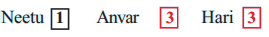How do we write the scores now?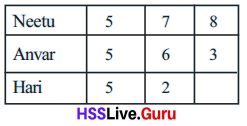They got into an argument about Hari’s score.
Hari said, since 3 cannot be subtracted from 2, his score should be written as 0.
Not so, said Anvar; Hari has lost and must quit the game;
Neetu and himself will continue.

No, said Neetu; Han can continue, but 1 will be subtracted from what he gets in the next round.
They all agreed and decided to write, “Subtract 1” in Hari’s column.
But then, asked Anvar, why not shorten it as just -1? All agreed on this also.Han was saved in the next round.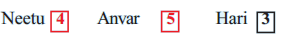Can you write their scores now?Hart got 3 subtracting the earlier debt of 1, his scores now is 2.
5 cannot be subtracted from 3
As with Han in the earlier round, they decided Anvar should subtract from the number he gets next.
Subtract how much?
“Subtract 2” can be written -2 as before.In the fourth round, they got these cards: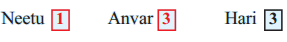Can you write the scores now?Explanation:
Score points of Neetu = Points scored in fourth level by Neetu – Points of card taken
= 4 – 1
= 3.
Score points of Anwar = Points scored in fourth level by Anwar – Points of card taken
= – 2 – 3
= -5.
Score points of Hari = Points scored in fourth level by Anwar + Points of card taken
= 2 + 3
= 5.Below Zero Textbook Page No. 180

In the card game when 3 had to be subtracted from 2, it was written as -1. Let’s write this operation as
2 – 3 = -1
What does this mean?
2 subtracted from 2 gives 0. Here we have to subtract 3 and so has to subtract 1 again. This we write as -1. That
0 – 1 = -1
Similarly, how was 5 subtracted from 3?
3 subtracted from 3 is 0; what more should be subtracted?
0 – 2 = -2
Numbers written with a minus sign like these are called negative numbers.
Let’s look at another problem.

An examination has 25 questions. Each correct answer gets 2 marks; for each wrong answer, 1 mark is taken away.
For example, if 19 answers are correct and 6 wrong, the total marks got is
(19 × 2) – 6 = 32
What if it is the other way round?
The 6 correct answers get (6 × 2) = 12 marks.
For the 19 wrong answers, 19 marks are reduced. So the total marks got is
12 – 19
How do we compute this?
12 subtracted from 12 gives 0, what more should be subtracted?
19 – 12 = 7

Thus
12 – 19 = 0 – 7 = – 7
Sometimes we need negative fractions also. Look at this problem.
An examination has 10 questions. Each correct answer gets 1 mark, and for each wrong answer, $$\frac{1}{2}$$ mark is reduced.
If one got only 3 answers right, what would be his total marks?
For the three correct answers, he would get 3 marks; and for the seven wrong answers, half of 7, that is 3$$\frac{1}{2}$$, would be subtracted.
3 subtracted from 3 makes 0; and $$\frac{1}{2}$$ more should be reduced.
So total marks is
3 – 3$$\frac{1}{2}$$ = 0 – $$\frac{1}{2}$$ = –$$\frac{1}{2}$$
How much marks for someone getting just 1 question right?
1 – 4$$\frac{1}{2}$$
How do we compute this?
1 – 1 = 0
What more to be subtracted?
4$$\frac{1}{2}$$ – 1 = 3$$\frac{1}{2}$$
So, we find
1 – 4$$\frac{1}{2}$$ = 0 – 3$$\frac{1}{2}$$ = -3$$\frac{1}{2}$$
When we start using negative numbers also, the numbers like 1, 2, 1$$\frac{1}{2}$$ (which are not negative) are called positive numbers.
What about 0? It’s neither positive, nor negative.
To subtract a larger positive number from a smaller positive number, we first go to zero and then subtract from zero. Can’t we do this directly instead?
Yes, we can do it directly by subtracting a large positive number from a smaller positive number, you get a negative number.

Explanation:
The rules for subtraction are similar to those of addition. For two positive numbers, if the first number is larger than the second, then the result is another positive number. If you subtract a large positive number from a smaller positive number, you get a negative number.

Let’s have another look at the computations done so far:
2 – 3 = -1                3 – 2 = 1
3 – 5 = -2               5 – 3 = 2
12 – 19 = -7           19 – 12 = 7
3 – 3$$\frac{1}{2}$$ = –$$\frac{1}{2}$$        3$$\frac{1}{2}$$ – 3 = $$\frac{1}{2}$$
1 – 4$$\frac{1}{2}$$ = -3$$\frac{1}{2}$$       4$$\frac{1}{2}$$ – 1 = 3$$\frac{1}{2}$$

What do we see from these?

For positive numbers, the larger subtracted from the smaller is the negative of the smaller subtracted from the larger.
We can write this using algebra also:
For any two positive numbers x, y if x < y then
x – y = -(y – x)

Now try these problems:

• 4 – 9
9 subtracted from 4, we get -5.

Explanation:
x = 4
y = 9
=> x < y
=> x – y = -(y – x)
=> 4 – 9 = -(9 – 4)
=> 4 – 9 = -(5)
=> 4 – 9 = -5.

• 14 – 29
29 subtracted from 14, we get -5.

Explanation:
x = 14
y = 29
=> x < y
=> 14 – 29 = -(y – x)
=> 14 – 29 = -(29 – 14)
=> 14 – 29 = -(15)
=> 14 – 29 = -15.

• $$\frac{1}{2}$$ – $$\frac{3}{4}$$
$$\frac{3}{4}$$ is subtracted from $$\frac{1}{2}$$, we get $$\frac{1}{4}$$

Explanation:
x = $$\frac{1}{2}$$
y = $$\frac{3}{4}$$
=> $$\frac{1}{2}$$ < $$\frac{3}{4}$$
=> $$\frac{1}{2}$$ – $$\frac{3}{4}$$ = -($$\frac{3}{4}$$– $$\frac{1}{2}$$)
=> $$\frac{1}{2}$$ – $$\frac{3}{4}$$ = -{[ 3 – 1(2)] ÷ 4}
=> $$\frac{1}{2}$$ – $$\frac{3}{4}$$ = -[(3 – 2) ÷ 4]
=> $$\frac{1}{2}$$ – $$\frac{3}{4}$$ = -($$\frac{1}{4}$$)
=> $$\frac{1}{2}$$ – $$\frac{3}{4}$$ = –$$\frac{1}{4}$$

• 5 – 10
10 subtracted from 5, we get – 5.

Explanation:
x = 5
y = 10
=> x < y
=> 5 – 10 = -(10 – 5)
=> 5 – 10 = -(5)
=> 5 – 10 = – 5.

• 25 – 65
65 subtracted from 25, we get -40.

Explanation:
x = 25
y = 65
=> x < y
=> 25 – 65 = -(65 – 25)
=> 25 – 65 = -(40)
=> 25 – 65 = – 40.

• $$\frac{1}{3}$$ – $$\frac{1}{2}$$
$$\frac{1}{2}$$ subtracted from $$\frac{1}{3}$$, we get $$\frac{1}{6}$$

Explanation:
x = $$\frac{1}{3}$$
y = $$\frac{1}{2}$$
=> $$\frac{1}{3}$$ < $$\frac{1}{2}$$
=> $$\frac{1}{3}$$ – $$\frac{1}{2}$$ = -($$\frac{1}{2}$$ – $$\frac{1}{3}$$)
=> $$\frac{1}{3}$$ – $$\frac{1}{2}$$ = – [(1 × 3) – (1 × 2) ÷ 6]
=> $$\frac{1}{3}$$ – $$\frac{1}{2}$$ = – [(3 – 2) ÷ 6]
=> $$\frac{1}{3}$$ – $$\frac{1}{2}$$ = – ($$\frac{1}{6}$$)
=> $$\frac{1}{3}$$ – $$\frac{1}{2}$$ = – $$\frac{1}{6}$$In the game of number cards when we say one’s score is -2, it means 2 is to be subtracted from the next card he
draws. So, if he draws a black 2 next, his score is
2 – 2 = 0
Adding 2 when the score is -2 can be written
-2 + 2
That is,
-2 + 2 = 2 – 2 = 0
In an exam of 10 questions, each correct answer is given 1 mark and for each wrong answer, 1 mark is subtracted.

If one gets the first 5 questions right and the next 5 wrong, what would be his total marks?
From the 5 marks for the correct answers, 5 marks are subtracted due to the wrong answers, so that total mark is
0.
If we do this in the order of the answers, we can write the total marks as – 5 + 5. Thus
-5 + 5 = 5 – 5 = 0
What if the first 4 answers are wrong and the next 6 right? We can write it as – 4 + 6. If from the 6 marks for the right answers, 4 marks are subtracted because of the wrong answers, it would give 6 – 4 = 2. So,
– 4 + 6 = 6 – 4 = 2
If the first 6 are wrong and the next 4 right?
The total marks can be written – 6 + 4.
From the 4 marks for the right answers, 6 marks are subtracted because of the wrong answers, it would give 4 – 6 = – 2. So,
– 6 + 4 = 4 – 6 = – 2
In an exam of 10 questions, each right answer gets 1 mark 1 and $$\frac{1}{2}$$ mark is subtracted for each wrong answer.

If one gets only the last 3 answers right, what would be his total marks?
We have already seen that in this case, the total marks is
3 – 3$$\frac{1}{2}$$ = –$$\frac{1}{2}$$. If marks are computed in the order of the answers, we can say that the total marks is -3$$\frac{1}{2}$$ + 3 also.
That is,
-3$$\frac{1}{2}$$ + 3 = 3 – 3$$\frac{1}{2}$$ = –$$\frac{1}{2}$$
Let’s look at all these computations together:
-2 + 2 = 2 – 2 = 0
-5 + 5 = 5 – 5 = 0
-4 + 6 = 6 – 4 = 2
-6 + 4 = 4 – 6 = -2
-3$$\frac{1}{2}$$ + 3 = 3 – 3$$\frac{1}{2}$$ = –$$\frac{1}{2}$$

What do we see from these?
Adding to the negative of a positive number, a second positive number means subtracting the first number from the second number.

In the language of algebra, this can be put this way:

For any two positive numbers x and y
– x + y = y – x

Now try these problems:

• – 4 + 9
9 subtracted from – 4, we get 5.

Explanation:
Two positive numbers x and y
– x + y = y – x
=> x = -4 ; y = 9
=> – 4 + 9 = 9 – 4
=> – 4 + 9 = 5.

• – 15 + 8
8 added to  – 15, we get -7.

Explanation:
Two positive numbers x and y
– x + y = y – x
=> x = -15 ; y = 8
=> – 15 + 8 = 8 – 15
=> – 15 + 8 = -7.

• –$$\frac{1}{2}$$ + $$\frac{3}{4}$$
$$\frac{3}{4}$$ added to –$$\frac{1}{2}$$, we get $$\frac{1}{4}$$.

Explanation:
Two positive numbers x and y
– x + y = y – x
=> x = –$$\frac{1}{2}$$ ; y = $$\frac{3}{4}$$
=> –$$\frac{1}{2}$$ + $$\frac{3}{4}$$ = $$\frac{3}{4}$$ – $$\frac{1}{2}$$
=> –$$\frac{1}{2}$$ + $$\frac{3}{4}$$ = {[(3 – (1 × 2)] ÷ 4}
=> –$$\frac{1}{2}$$ + $$\frac{3}{4}$$ = [(3 – 2) ÷ 4]
=> –$$\frac{1}{2}$$ + $$\frac{3}{4}$$ = $$\frac{1}{4}$$

• -9 + 4
4 added to -9, we get -5.

Explanation:
Two positive numbers x and y
– x + y = y – x
=> x = -9 ; y = 4
=> – 9 + 4 = 4 – 9
=> – 9 + 4 = -5.

• -8 + 15
15 added to -8, we get 7.

Explanation:
Two positive numbers x and y
– x + y = y – x
=> x = -8 ; y = 15
=> – 8 + 15 = 15 – 8
=> – 8 + 15 = 7.

• –$$\frac{3}{4}$$ + $$\frac{1}{2}$$
$$\frac{1}{2}$$ added to –$$\frac{3}{4}$$, we get –$$\frac{1}{4}$$

Explanation:
Two positive numbers x and y
– x + y = y – x
=> x = –$$\frac{3}{4}$$ ; y = $$\frac{1}{2}$$
=> –$$\frac{3}{4}$$ + $$\frac{1}{2}$$ = $$\frac{1}{2}$$ –$$\frac{3}{4}$$
=> –$$\frac{3}{4}$$ + $$\frac{1}{2}$$ = [(1 × 2) – 3] ÷ 4]
=> –$$\frac{3}{4}$$ + $$\frac{1}{2}$$ = [(2 – 3) ÷ 4]
=> –$$\frac{3}{4}$$ + $$\frac{1}{2}$$ = –$$\frac{1}{4}$$Subtracting again Textbook Page No. 184

In an exam where 1 mark is subtracted for each wrong answer, if the first two answers are wrong, what would be the total marks then?
If the next answer also is wrong?
Since 3 answers are wrong, the marks then is -3.

We can look at this in another manner. With the first two wrong answers, marks is – 2, with the next also wrong, 1 more mark should be subtracted, That is,
– 2 – 1 = – 3
What if the next two answers are also wrong?
5 answers are wrong; so – 5 marks. Looking at it another way, 2 subtracted from – 3; that is – 3 – 2. We can write this as
– 3 – 2 = – 5
So, then how much – 5 – 3 ?
– 5 means 5 less then 0; if it is still 3 less, how much less than 0 altogether?

That is,
– 5 – 3 = – (5 + 3) = – 8
Can’t we calculate – 5 – 7 like this?
– 5 – 7 = – (5 + 7) = – 12
We can state this as a general principle:
Subtracting a positive number from the negative of a positive number, we get the negative ofthe sum of these positive numbers.

How do we write it using algebra?

For any positive numbers x and y,
-x – y = – (x + y)

Do the following problems using this.

Question 1.
-1 – 1
1 subtracted from -1, we get -2.

Explanation:
-x – y = – (x + y)
=> x = -1; y = 1.
=> -1 – 1 = -(1 + 1)
=> -1 – 1 = -(2)
=> -1 – 1 = -2.

Question 2.
-7 – 8
8 subtracted from -7, we get -15.

Explanation:
-x – y = – (x + y)
=> x = -7; y = 8.
=> -7 – 8 = -(8 + 7)
=> -7 – 8 = -(15)
=> -7 – 8 = -15.

Question 3.
–$$\frac{1}{2}$$ – $$\frac{1}{4}$$
$$\frac{1}{4}$$ subtracted from –$$\frac{1}{2}$$, we get –$$\frac{3}{4}$$

Explanation:
-x – y = – (x + y)
=> x = –$$\frac{1}{2}$$; y = $$\frac{1}{4}$$.
=> –$$\frac{1}{2}$$ – $$\frac{1}{4}$$ = -($$\frac{1}{2}$$ + $$\frac{1}{4}$$)
=> –$$\frac{1}{2}$$ – $$\frac{1}{4}$$ = -{[(1× 2) + 1] ÷ 4}
=> –$$\frac{1}{2}$$ – $$\frac{1}{4}$$ =[(2 + 1) ÷ 4]
=> –$$\frac{1}{2}$$ – $$\frac{1}{4}$$ = -($$\frac{3}{4}$$)
=> –$$\frac{1}{2}$$ – $$\frac{1}{4}$$ = –$$\frac{3}{4}$$

Question 4.
-2 – 2
2 subtracted from -2,we get -4.

Explanation:
-x – y = – (x + y)
=> x = -2; y = 2.
=> -2 – 2 = -(2 + 2)
=> -2 – 2 = -(4)
=> -2 – 2 = -4.

Question 5.
-8 – 7
7 subtracted from -8, we get -15.

Explanation:
-x – y = – (x + y)
=> x = -8; y = 7.
=> -8 – 7 = -(8 + 7)
=> -8 – 7 = -(15)
=> -8 – 7 = -15.

Question 6.
-2$$\frac{1}{2}$$ – 1$$\frac{1}{2}$$
1$$\frac{1}{2}$$ subtracted from -2$$\frac{1}{2}$$, we get -4.

Explanation:
-x – y = – (x + y)
=> x = -2$$\frac{1}{2}$$ ; y = 1$$\frac{1}{2}$$.
=> -2$$\frac{1}{2}$$ – 1$$\frac{1}{2}$$ = -(2$$\frac{1}{2}$$ + 1$$\frac{1}{2}$$)
=> -2$$\frac{1}{2}$$ – 1$$\frac{1}{2}$$ = – {[(2 × 2) + 1] ÷2} + {[(1 × 2) + 1] ÷2}
=> -2$$\frac{1}{2}$$ – 1$$\frac{1}{2}$$ = -[(4 + 1) ÷ 2] + [(2 + 1) ÷ 2]
=> -2$$\frac{1}{2}$$ – 1$$\frac{1}{2}$$ = -{$$\frac{5}{2}$$ + $$\frac{3}{2}$$ }
=> -2$$\frac{1}{2}$$ – 1$$\frac{1}{2}$$ = -[(5 + 3) ÷ 2]
=> -2$$\frac{1}{2}$$ – 1$$\frac{1}{2}$$ = –$$\frac{8}{2}$$
=> -2$$\frac{1}{2}$$ – 1$$\frac{1}{2}$$ = –$$\frac{4}{1}$$
=> -2$$\frac{1}{2}$$ – 1$$\frac{1}{2}$$ = -4.

Question 7.
8 – 12
12 subtracted from 8, we get -4.

Explanation:
-x – y = – (x + y)
=> x = 8; y = 12.
=> 8 – 12 = -(-8 + 12)
=> 8 – 12 = -(4)
=> 8 – 12 = -4.

Question 8.
-10 – 4
4 subtracted from -10, we get -14.

Explanation:
-x – y = – (x + y)
=> x = -10; y = 4.
=> -10 – 4 = -(10 + 4)
=> -10 – 4 = -(14)
=> -10 – 4 = -14.

Question 9.
1$$\frac{1}{2}$$ – 7$$\frac{1}{2}$$
7$$\frac{1}{2}$$ subtracted from 1$$\frac{1}{2}$$, we get 6.

Explanation:
-x – y = – (x + y)
=> x = 1$$\frac{1}{2}$$ ; y = 7$$\frac{1}{2}$$.
=> 1$$\frac{1}{2}$$ – $$\frac{1}{2}$$ = -(1$$\frac{1}{2}$$ + 7$$\frac{1}{2}$$)
=> 1$$\frac{1}{2}$$ – 7$$\frac{1}{2}$$ = -{[(1 × 2) + 1] ÷ 2} + {[(7 × 2) + 1] ÷ 2}
=> 1$$\frac{1}{2}$$ – 7$$\frac{1}{2}$$ = -[-(2 + 1) ÷ 2] + [(14 + 1) ÷ 2]
=> 1$$\frac{1}{2}$$ -7 $$\frac{1}{2}$$ =- {-$$\frac{3}{2}$$ + $$\frac{15}{2}$$}
=> 1$$\frac{1}{2}$$ – 7$$\frac{1}{2}$$ = – [(-3 + 15)÷ 2]
=> 1$$\frac{1}{2}$$ – 7$$\frac{1}{2}$$ = -($$\frac{12}{2}$$
=> 1$$\frac{1}{2}$$ – 7$$\frac{1}{2}$$ = -($$\frac{6}{1}$$
=> 1$$\frac{1}{2}$$ – 7$$\frac{1}{2}$$ = -6.

Question 10.
-25 – 3$$\frac{1}{2}$$
3$$\frac{1}{2}$$ subtracted from -25, we get -28$$\frac{1}{2}$$

Explanation:
-x – y = – (x + y)
=> x = -25; y = 3$$\frac{1}{2}$$.
=> -25 – 3$$\frac{1}{2}$$ = -(25 + 3$$\frac{1}{2}$$)
=> -25 – 3$$\frac{1}{2}$$ = -(25 + {[(3 × 2) + 1] ÷ 2}
=> -25 – 3$$\frac{1}{2}$$ = -(25 +[(6 + 1) ÷ 2])
=> -25 – 3$$\frac{1}{2}$$ = -(25 + $$\frac{7}{2}$$
=> -25 – 3$$\frac{1}{2}$$ = -[(50 + 7) ÷ 2]
=> -25 – 3$$\frac{1}{2}$$ = –$$\frac{57}{2}$$
=> -25 – 3$$\frac{1}{2}$$ = -28$$\frac{1}{2}$$

Question 11.
-8 + 8
8 added to -8, we get 0.

Explanation:
-x – y = – (x + y)
=> x = -8; y = 8.
=> -8 + 8 = -(8 – 8)
=> -8 + 8 = -(0)
=> -8 + 8 = 0.

Question 12.
-10 + 20
20 added to -10, we get 10.

Explanation:
-x – y = – (x + y)
=> x = -10; y = 20.
=> -10 + 20 = -(10 – 20)
=> -10 + 20 = -(-10)
=>-10 + 20 = 10.

Question 13.
– 3$$\frac{1}{2}$$ + 3$$\frac{1}{2}$$
3$$\frac{1}{2}$$ added to – 3$$\frac{1}{2}$$, we get 0.

Explanation:
-x – y = – (x + y)
=> x = – 3$$\frac{1}{2}$$ ; y = 3$$\frac{1}{2}$$.
=> – 3$$\frac{1}{2}$$ + (3$$\frac{1}{2}$$) = -( 3$$\frac{1}{2}$$ – 3$$\frac{1}{2}$$)
=> – 3$$\frac{1}{2}$$ + (3$$\frac{1}{2}$$) = -(0)
=> – 3$$\frac{1}{2}$$ + (3$$\frac{1}{2}$$) = 0.

Question 14.
-20 + 40
40 added to -20, we get 20.

Explanation:
-x – y = – (x + y)
=> x = -20; y = 40.
=> -20 + 40 = -(20 – 40)
=> -20 + 40 = -(-20)
=> -20 + 40 = 20.

Question 15.
-7 + 4
4 added to -7, we get -3.

Explanation:
-x – y = – (x + y)
=> x = -7; y = 4.
=> -7 + 4 = -(7 – 4)
=> -7 + 4 = -(3)
=> -7 + 4 = -3.

Question 16.
-4$$\frac{1}{2}$$ + 5$$\frac{1}{2}$$
5$$\frac{1}{2}$$ added to -4$$\frac{1}{2}$$, we get 1.

Explanation:
-x – y = – (x + y)
=> x = -4$$\frac{1}{2}$$; y = 5$$\frac{1}{2}$$.
=> -4$$\frac{1}{2}$$ + 5$$\frac{1}{2}$$ = -(4$$\frac{1}{2}$$ – 5$$\frac{1}{2}$$)
=> -4$$\frac{1}{2}$$ + 5$$\frac{1}{2}$$ = -{[(4 × 2) + 1] ÷ 2} – {[(5 × 2) + 1] ÷ 2}
=> -4$$\frac{1}{2}$$ + 5$$\frac{1}{2}$$ = -{[(8 + 1) ÷ 2] – [(10 + 1) ÷ 2]}
=> -4$$\frac{1}{2}$$ + 5$$\frac{1}{2}$$ = -($$\frac{9}{2}$$ – $$\frac{11}{2}$$}
=> -4$$\frac{1}{2}$$ + 5$$\frac{1}{2}$$ = -[(9 – 11) ÷ 2]
=> -4$$\frac{1}{2}$$ + 5$$\frac{1}{2}$$ = – [-($$\frac{2}{2}$$]
=> -4$$\frac{1}{2}$$ + 5$$\frac{1}{2}$$ = -(-$$\frac{1}{1}$$]
=> -4$$\frac{1}{2}$$ + 5$$\frac{1}{2}$$ =- (-1)
=> -4$$\frac{1}{2}$$ + 5$$\frac{1}{2}$$ = 1.

Question 17.
-12$$\frac{1}{2}$$ + $$\frac{1}{2}$$
$$\frac{1}{2}$$ added to -12$$\frac{1}{2}$$, we get 12.
=> x = -12$$\frac{1}{2}$$ ; y = $$\frac{1}{2}$$.
=> -12$$\frac{1}{2}$$ + $$\frac{1}{2}$$ = -(12$$\frac{1}{2}$$ – $$\frac{1}{2}$$)
=> -12$$\frac{1}{2}$$ + $$\frac{1}{2}$$ = -{[(12 × 2) + 1] ÷ 2} – $$\frac{1}{2}$$}
=> -12$$\frac{1}{2}$$ + $$\frac{1}{2}$$ = – [(24 + 1) ÷ 2] – $$\frac{1}{2}$$}
=> -12$$\frac{1}{2}$$ + $$\frac{1}{2}$$ = – ($$\frac{25}{2}$$ – $$\frac{1}{2}$$}
=> -12$$\frac{1}{2}$$ + $$\frac{1}{2}$$ = -[(25 – 1) ÷ 2]
=> -12$$\frac{1}{2}$$ + $$\frac{1}{2}$$ =.-($$\frac{24}{2}$$)
=> -12$$\frac{1}{2}$$ + $$\frac{1}{2}$$ = – $$\frac{12}{1}$$
=> -12$$\frac{1}{2}$$ + $$\frac{1}{2}$$ = -12.